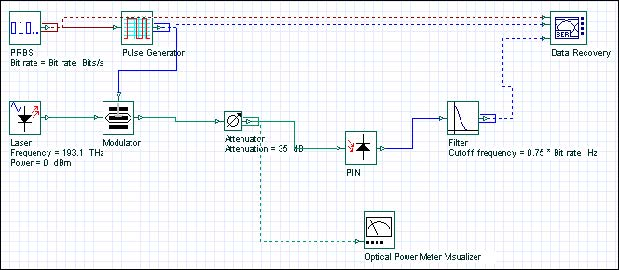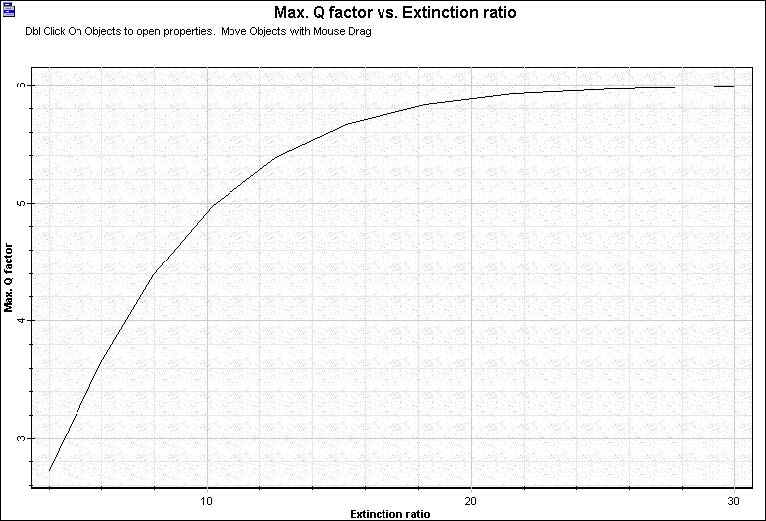A simple source of power penalty is related to the energy carried by 0 bits. Some power is emitted by transmitters even in the off-state. Sensitivity Degradation – ER.osd includes an external modulated laser where you can specify the extinction ratio at the modulator (see Figure 1).Figure 1: Sensitivity Degradation – ER

In this project we vary the value of the ER and we calculate the Q factor at the receiver (see Figure 2).Figure 2: Q factor x ER